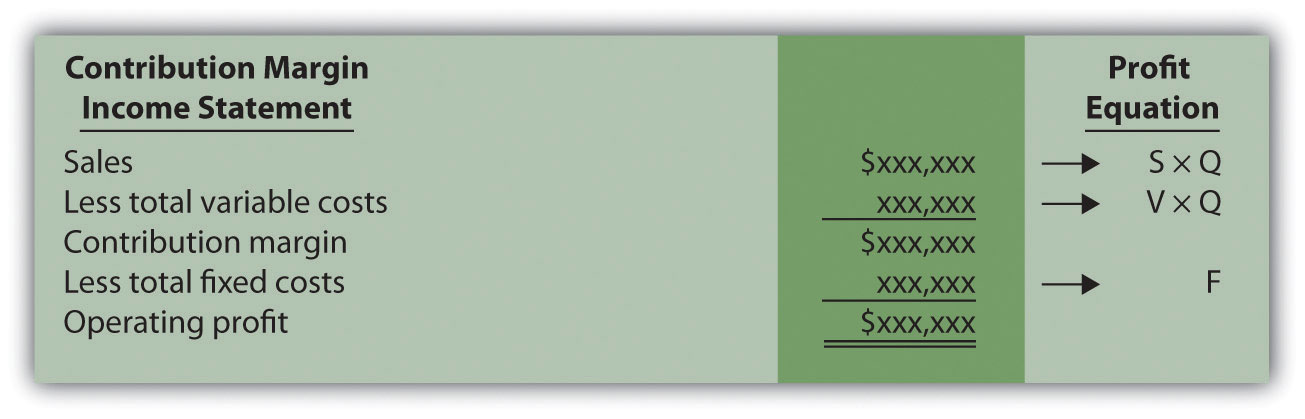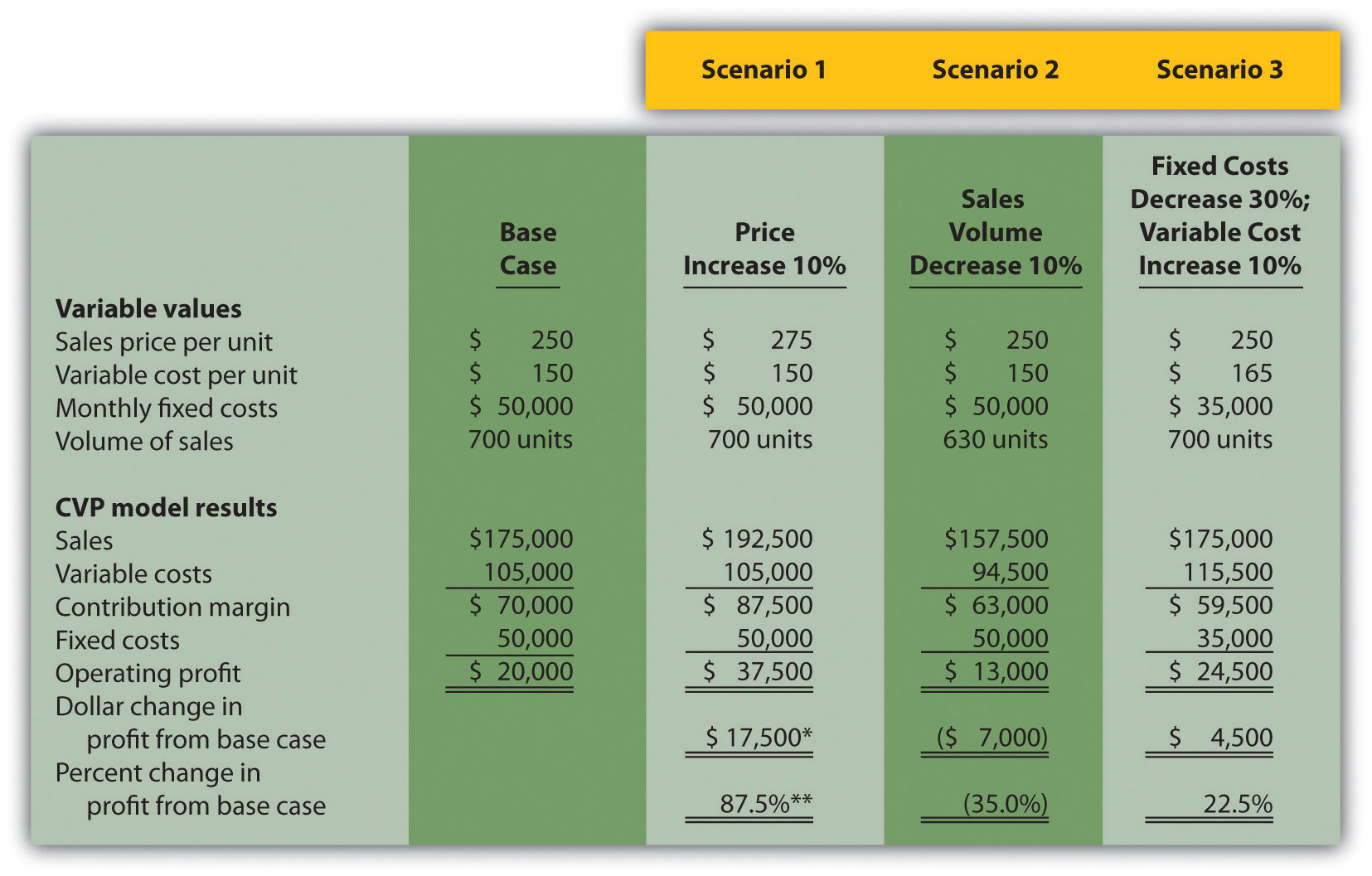## Unit 4 Study Guide: Cost-Volume-Profit Analysis

### 4a: Perform cost-volume-profit analysis for single-product companies.

The contribution margin describes the amount of sales that will contribute to fixed costs. Managers calculate the contribution margin by subtracting variable costs from sales. Managers deduct their fixed costs from their contribution margin to calculate operating profit or loss. Any money that remains after fixed costs are covered contributes to the company profits.

• What is the relationship between the profit equation and the contribution margin income statement?
• How does a variable cost differ from a fixed cost?

Review these concepts in Cost-Volume-Profit Analysis for Single-Product Companies from Managerial Accounting.

Review the relationship between the contribution margin income statement and the profit equation in Figure 6.1.

Figure 6.1 Comparison of Contribution Margin Income Statement with Profit EquationThe break-even point indicates the point where the amount of sales (in terms of units sold or money earned) covers all variable and fixed costs (and results in zero profits).

• Calculate the break-even point in units using the formula (hint: set the target profit to zero):

Quantity = Fixed Costs + Target Profit / Sales Price – Variable Costs

• Calculate the contribution margin per unit using the formula:

Sales Price Per Unit – Variable Costs Per Unit

• Calculate the calculation margin ratio using the formula:

Contribution Margin Per Unit / Sales Price Per Unit

• Calculate the break-even point in sales dollars using the formula:

Fixed Costs / Contribution Margin Ratio

Most companies want to know how many units they need to sell (i.e. the sales volume they need to reach) to meet a targeted profit amount. Managers use the target profit in equations to calculate the number of units the company must sell (or the sales volume they must reach).

• Calculate the quantity of units a company must sell to reach the target profit using the formula:

Quantity = Fixed Costs + Target Profit / Sales Price – Variable Costs

• Calculate the level of sales volume a company must reach to earn the target profit using the formula:
Fixed Costs + Target Profit / Contribution Margin Ratio

The margin of safety equals the amount of sales (in units or dollars) that can decrease before the company experiences an overall loss.

• Calculate the margin of safety in units using the formula:

Margin of Safety (in units) = Projected Sales (in units) − Break-Even Sales (in units)

• Calculate the margin of safety in sales dollars using the formula:

Margin of Safety (in sales dollars) = Projected Sales (in sales dollars) − Break-even Sales dollars

Review cost-volume-profit (CVP) analysis in Cost-Volume-Profit Analysis—Part 1 by Tony Bell.

### 4b: Use sensitivity analysis to determine how changes in the cost-volume-profit equation affect profit.

Managers use a "what-if" or sensitivity analysis, to plan their future earnings. For example, a manager might consider what would happen to the break-even point and/or operating profits if fixed costs increased by \$10,000.

• Describe the process of changing variables in cost-volume-profit (CVP) models to determine changes in profits.

Review these concepts in Using Cost-Volume-Profit Models for Sensitivity Analysis from Managerial Accounting.

Review sensitivity analysis in Figure 6.6.

Figure 6.6 Sensitivity Analysis for Snowboard Company.### 4c: Describe how cost structure affects cost-volume-profit sensitivity analysis.

Manufacturing firms determine their cost structure by analyzing the proportion of fixed and variable costs to their total costs.

• Explain what it means to have a high operating leverage.

Review the effect cost structure has on CVP sensitivity analysis in Impact of Cost Structure on Cost-Volume-Profit Analysis from Managerial Accounting.

### 4d: Use an alternative form of contribution margin when faced with a resource constraint.

A constraint occurs when companies have limited resources in a particular area.

• Describe the benefit of analyzing contribution margins with units of constraint when making decisions.

Review the process of analysis with resource constraints in Using a Contribution Margin When Faced with Resource Constraints from Managerial Accounting.

### Unit 4 Vocabulary

• Break-even point (sales dollars)
• Break-even point (units)
• Contribution margin income statement
• Contribution margin per unit
• Contribution margin per unit of constraint
• Contribution margin ratio
• Cost structure
• CVP graph
• Fixed cost
• Margin of safety
• Operating leverage
• Profit equation
• Sensitivity analysis
• Target profit (units)
• Target profits (sales dollars)
• Variable cost